# PSAT Math : How to add rational expressions with different denominators

## Example Questions

### Example Question #1 : Rational Expressions

Simplify the expression.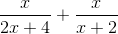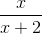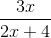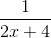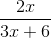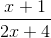Explanation:

To add rational expressions, first find the least common denominator. Because the denominator of the first fraction factors to 2(x+2), it is clear that this is the common denominator. Therefore, multiply the numerator and denominator of the second fraction by 2.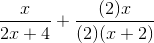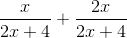This is the most simplified version of the rational expression.